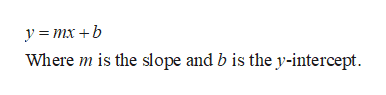Find an equation of the line that passes through the given points. (Let x be the independent variable and y be the dependent variable.)(2, 2) and (3, 7)

Question

Find an equation of the line that passes through the given points. (Let x be the independent variable and y be the dependent variable.)

(2, 2) and (3, 7)

Step 1

Given:

The line passes through the points (2,2) and (3,7).

The equation of the l...help_outlineImage Transcriptionclosey =mx +b Where m is the slope and b is the y-intercept fullscreen

Want to see the full answer?

See Solution

Want to see this answer and more?

Our solutions are written by experts, many with advanced degrees, and available 24/7

See Solution
Tagged in

Other